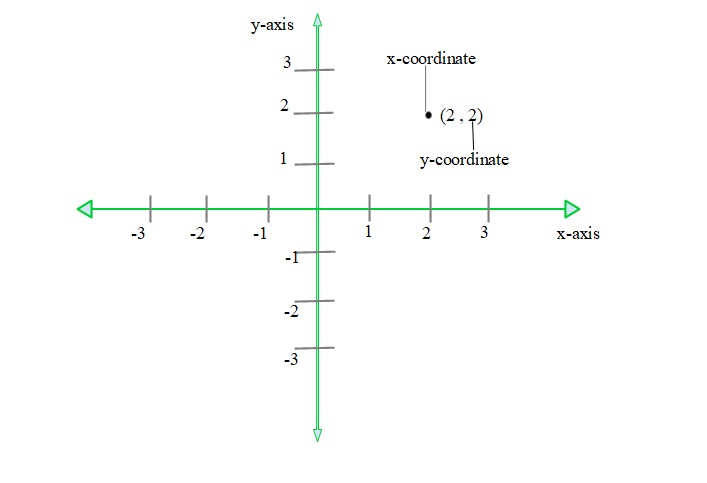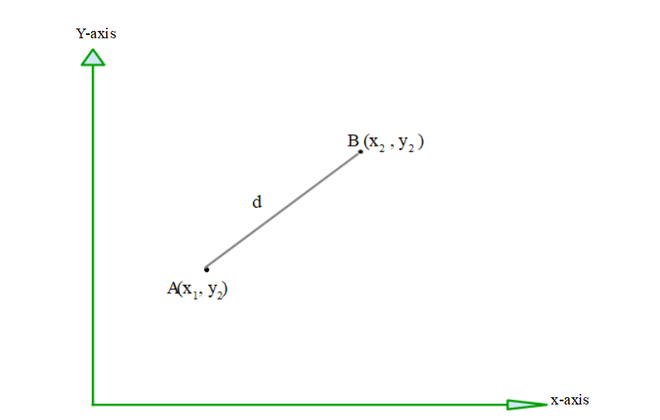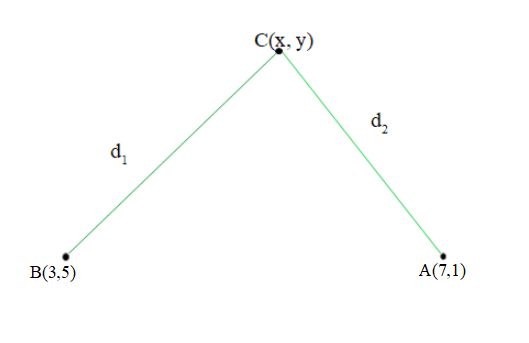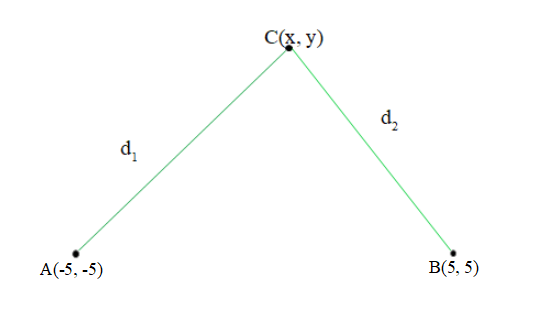# Find a relation between x and y such that the point (x, y) is equidistant from the points (7, 1) and (3, 5)

• Last Updated : 13 Jan, 2022

Mathematics is related to numbers and calculations. Mathematics is divided into different branches on the basis of the type of operations or calculations like algebra, geometry, arithmetic, etc. Geometry It is the branch of mathematics that deals with shape and their properties. Geometry that deals with points, lines, and planes in which coordinates are involved is called coordinate geometry.

### Coordinates

The location of any point on a plane can be expressed as (x, y) and these pairs are called the coordinates of that point, x is the horizontal value of a point on the plane. This value can also be called the x-coordinate or Abscissa, y is the vertical value of a point on the plane. This value can be called the y-coordinate or ordinate. In coordinate geometry, the point is represented on the cartesian plane.

### Cartesian plane

It is a plane formed by two perpendicular lines that is the x-axis (horizontal axis) and the y-axis (vertical axis). The position of a point in the Cartesian plane can be represented using the ordered pair (x, y).### Distance formula

This formula is used to calculate the distance between two points on a plane. In other words, it gives the length of the line segment which can be formed after joining two points. Let the two points be A and B, having coordinates to be (x1, y1) and (x2, y2) respectively. Then, the distance between two points is given as,Distance (d)=### Find the relation between x and y such that the point(x, y) is equidistant from (7, 1) and (3, 5).

Solution:

Equidistant means having equal distance. (x, y) is equidistant from point (7, 1) and (3, 5), which means distance of point (x, y) from both the point is equal.

Lets suppose A and B be the name of point (7, 1) and (3, 5) respectively and C be the point having coordinated (x, y).So, according to the question, d1 and d1 are equal.

CB = AC

Use distance formula to find d1 and d2 values.

• AC (Distance between (x, y) and (7, 1))• CB (Distance between (x, y) and (3, 5))As, CB = ACSquaring both sides,

(x2 – 14x + y2 – 2y + 50) = (x2 – 6x + y2 – 10y + 34)

x2 – x2 + y2 – y2 – 14x + 6x – 2y + 10y = 34 – 50

-8x + 8y = -16

8x – 8y = 16

x – y = 2

x = 2 + y

Hence, relation between x and y such that the point(x, y) is equidistant from (7, 1) and (3 , 5) is x = 2 + y

### Sample Problems

Question 1: Find the relation between x and y such that the point(x, y) is equidistant from (-5, -5) and (5, 5).

Solution:

Equidistant means having equal distance. (x, y) is equidistant from point (-5, -5) and (5, 5), which means distance of point (x, y) from both the point is equal.

Lets suppose A and B be the name of point (-5, -5) and (5, 5) respectively and C be the point having coordinated (x, y).So, according to the question, d1 and d2 are equal.

CB = AC

Use distance formula to find d1 and d2 values.

• AC (Distance between (x , y) and (-5, -5))• CB (Distance between (x , y) and (5 , 5))As, CB = ACSquaring both sides,

(x2 – 10x + y2 – 10y + 50) = (x2 + 10x + y2 + 10y + 50)

x2 – x2 + y2 – y2 = 10x + 10x + 10y + 10y + 50 – 50

0 = 20x + 20y

x = -y

Hence, relation between x and y such that the point (x, y) is equidistant from (-5, -5) and (5, 5) is x = -y

Question 2: Find the relation between x and y such that the point (x, y) is equidistant from (-2, 0) and (2, 0).

Solution:

(x, y) is equidistant from point (-2, 0) and (2, 0), which means distance of point (x, y) from both the point is equal. Let’s suppose A and B be the name of point (-2, 0) and (2, 0) respectively and C be the point having coordinated (x, y). So, according to the question, CB and AC are equal.

CB = AC

Use distance formula to find the distances CB and AC.

• AC (Distance between (x, y) and (-2, -0))• CB (Distance between (x, y) and (2, 0))As, CB = AC

\sqrt(x^2-4x+4)+(y^2)  = \sqrt(x^2+ 4x + 4)+(y^2 )

Squaring both sides,

(x2 – 4x + 4) + (y2) = (x2 + 4x + 4) + (y2)

x2 – x2 + y2 – y2 = 4x + 4x  + 4 – 4

0 = 8x

x = 0

x = 0  means y-axis.

Therefore, all the points that lie on y-axis is equidistant from point (-2, 0) and (2, 0).

Hence, relation between x and y such that the point (x, y) is equidistant from (-2, 0) and (2, 0) is x = 0

My Personal Notes arrow_drop_up
Recommended Articles
Page :